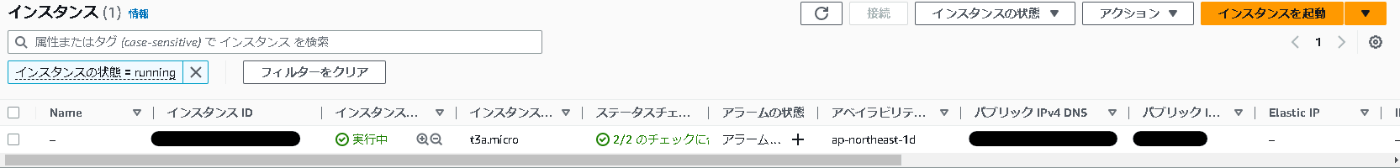🤖

# WSL2からTerraformでEC2インスタンスを立てるまで

2023/04/09に公開約9,900字

# はじめに

• 手順
• AWSCLIのインストール
• コンソールでアクセスキーの作成
• Terraformのインストール
• マニフェストの作成
• マニフェストのデプロイ

# AWSCLIの設定

## アクセスキーの作成

1. AWSのコンソールにルートユーザーでログインしてIAMサービスを選択
2. ユーザーから開発用のアカウントを選択
3. セキュリティ認証情報タブを選択して下にスクロール
4. アクセスキーを作成からCLI用のアクセスキーを作成する
• AWS CloudShellのサジェストをされるが、そのまま作成
5. アクセスキーとシークレットが作成される
6. `ダウンロードCSV`でローカルにCredentialを保持しておく

## 環境変数にアクセスキーを設定する

プロファイルを作っておきたかったので下記コマンドでプロファイルを作成
※プロファイルを作成後に環境変数に設定する必要があるため注意

``````mazainu@mazai:~/terraform\$ aws configure --profile development
AWS Access Key ID [None]: xxxxxxxxxxxxx
AWS Secret Access Key [None]: yyyyyyyyyyyyy
Default region name [None]: ap-northeast-1
Default output format [None]: json
mazainu@mazai:~/terraform\$ export AWS_PROFILE=development
``````

プロファイルを設定し忘れると下記のようなエラーになる

``````mazainu@mazai:~/terraform\$ terraform plan

Planning failed. Terraform encountered an error while generating this plan.

╷
│ Error: configuring Terraform AWS Provider: no valid credential sources for Terraform AWS Provider found.
│
│
│ AWS Error: failed to refresh cached credentials, no EC2 IMDS role found, operation error ec2imds: GetMetadata, request canceled, context deadline exceeded
│
│
│   with provider["registry.terraform.io/hashicorp/aws"],
│   on ec2.tf line 1, in provider "aws":
│    1: provider "aws" {
│
╵
``````

# Terraform

## 最小のマニフェストを作成

EC2インスタンスを作成する

• サイズ
• t3a.micro
• AMI
• ami-06e46074ae430fba6(Amazon Linux 2023 AMI)
• インスタンス名
• example

※リージョンによってAMIのIDが違うので注意です。

``````provider "aws" {
region = "ap-northeast-1"
}

resource "aws_instance" "example" {
ami           = "ami-06e46074ae430fba6"
instance_type = "t3a.micro"
}
``````

## 動作を確認

### コンポーネントをインストール

``````mazainu@mazai:~/terraform\$ terraform init

Initializing the backend...

Initializing provider plugins...
- Installing hashicorp/aws v4.61.0...
- Installed hashicorp/aws v4.61.0 (signed by HashiCorp)

Terraform has created a lock file .terraform.lock.hcl to record the provider
selections it made above. Include this file in your version control repository
so that Terraform can guarantee to make the same selections by default when
you run "terraform init" in the future.

Terraform has been successfully initialized!

You may now begin working with Terraform. Try running "terraform plan" to see
any changes that are required for your infrastructure. All Terraform commands
should now work.

If you ever set or change modules or backend configuration for Terraform,
rerun this command to reinitialize your working directory. If you forget, other
commands will detect it and remind you to do so if necessary.
``````

### 差分を確認

``````mazainu@mazai:~/terraform\$ terraform plan

Terraform used the selected providers to generate the following execution plan. Resource actions are
indicated with the following symbols:
+ create

Terraform will perform the following actions:

# aws_instance.example will be created
+ resource "aws_instance" "example" {
+ ami                                  = "ami-06e46074ae430fba6"
+ arn                                  = (known after apply)
+ associate_public_ip_address          = (known after apply)
+ availability_zone                    = (known after apply)
+ cpu_core_count                       = (known after apply)
+ cpu_threads_per_core                 = (known after apply)
+ disable_api_stop                     = (known after apply)
+ disable_api_termination              = (known after apply)
+ ebs_optimized                        = (known after apply)
+ host_id                              = (known after apply)
+ host_resource_group_arn              = (known after apply)
+ iam_instance_profile                 = (known after apply)
+ id                                   = (known after apply)
+ instance_initiated_shutdown_behavior = (known after apply)
+ instance_state                       = (known after apply)
+ instance_type                        = "t3a.micro"
+ ipv6_address_count                   = (known after apply)
+ ipv6_addresses                       = (known after apply)
+ key_name                             = (known after apply)
+ monitoring                           = (known after apply)
+ outpost_arn                          = (known after apply)
+ password_data                        = (known after apply)
+ placement_group                      = (known after apply)
+ placement_partition_number           = (known after apply)
+ primary_network_interface_id         = (known after apply)
+ private_dns                          = (known after apply)
+ private_ip                           = (known after apply)
+ public_dns                           = (known after apply)
+ public_ip                            = (known after apply)
+ secondary_private_ips                = (known after apply)
+ security_groups                      = (known after apply)
+ source_dest_check                    = true
+ subnet_id                            = (known after apply)
+ tags_all                             = (known after apply)
+ tenancy                              = (known after apply)
+ user_data                            = (known after apply)
+ user_data_base64                     = (known after apply)
+ user_data_replace_on_change          = false
+ vpc_security_group_ids               = (known after apply)
}

Plan: 1 to add, 0 to change, 0 to destroy.

────────────────────────────────────────────────────────────────────────────────────────────────────

Note: You didn't use the -out option to save this plan, so Terraform can't guarantee to take exactly
these actions if you run "terraform apply" now.
``````

### インスタンスを起動

``````mazainu@mazai:~/terraform\$ terraform apply

Terraform used the selected providers to generate the following execution plan. Resource actions are
indicated with the following symbols:
+ create

Terraform will perform the following actions:

# aws_instance.example will be created
+ resource "aws_instance" "example" {
+ ami                                  = "ami-079a2a9ac6ed876fc"
+ arn                                  = (known after apply)
+ associate_public_ip_address          = (known after apply)
+ availability_zone                    = (known after apply)
+ cpu_core_count                       = (known after apply)
+ cpu_threads_per_core                 = (known after apply)
+ disable_api_stop                     = (known after apply)
+ disable_api_termination              = (known after apply)
+ ebs_optimized                        = (known after apply)
+ host_id                              = (known after apply)
+ host_resource_group_arn              = (known after apply)
+ iam_instance_profile                 = (known after apply)
+ id                                   = (known after apply)
+ instance_initiated_shutdown_behavior = (known after apply)
+ instance_state                       = (known after apply)
+ instance_type                        = "t3a.micro"
+ ipv6_address_count                   = (known after apply)
+ ipv6_addresses                       = (known after apply)
+ key_name                             = (known after apply)
+ monitoring                           = (known after apply)
+ outpost_arn                          = (known after apply)
+ password_data                        = (known after apply)
+ placement_group                      = (known after apply)
+ placement_partition_number           = (known after apply)
+ primary_network_interface_id         = (known after apply)
+ private_dns                          = (known after apply)
+ private_ip                           = (known after apply)
+ public_dns                           = (known after apply)
+ public_ip                            = (known after apply)
+ secondary_private_ips                = (known after apply)
+ security_groups                      = (known after apply)
+ source_dest_check                    = true
+ subnet_id                            = (known after apply)
+ tags_all                             = (known after apply)
+ tenancy                              = (known after apply)
+ user_data                            = (known after apply)
+ user_data_base64                     = (known after apply)
+ user_data_replace_on_change          = false
+ vpc_security_group_ids               = (known after apply)
}

Plan: 1 to add, 0 to change, 0 to destroy.

Do you want to perform these actions?
Terraform will perform the actions described above.
Only 'yes' will be accepted to approve.

Enter a value: yes

aws_instance.example: Creating...
aws_instance.example: Still creating... [10s elapsed]
aws_instance.example: Creation complete after 12s [id=i-0ea286663051408e3]

Apply complete! Resources: 1 added, 0 changed, 0 destroyed.
``````

このようにAWSのコンソールからインスタンスの起動ができていることが確認できました！### Discussionログインするとコメントできます